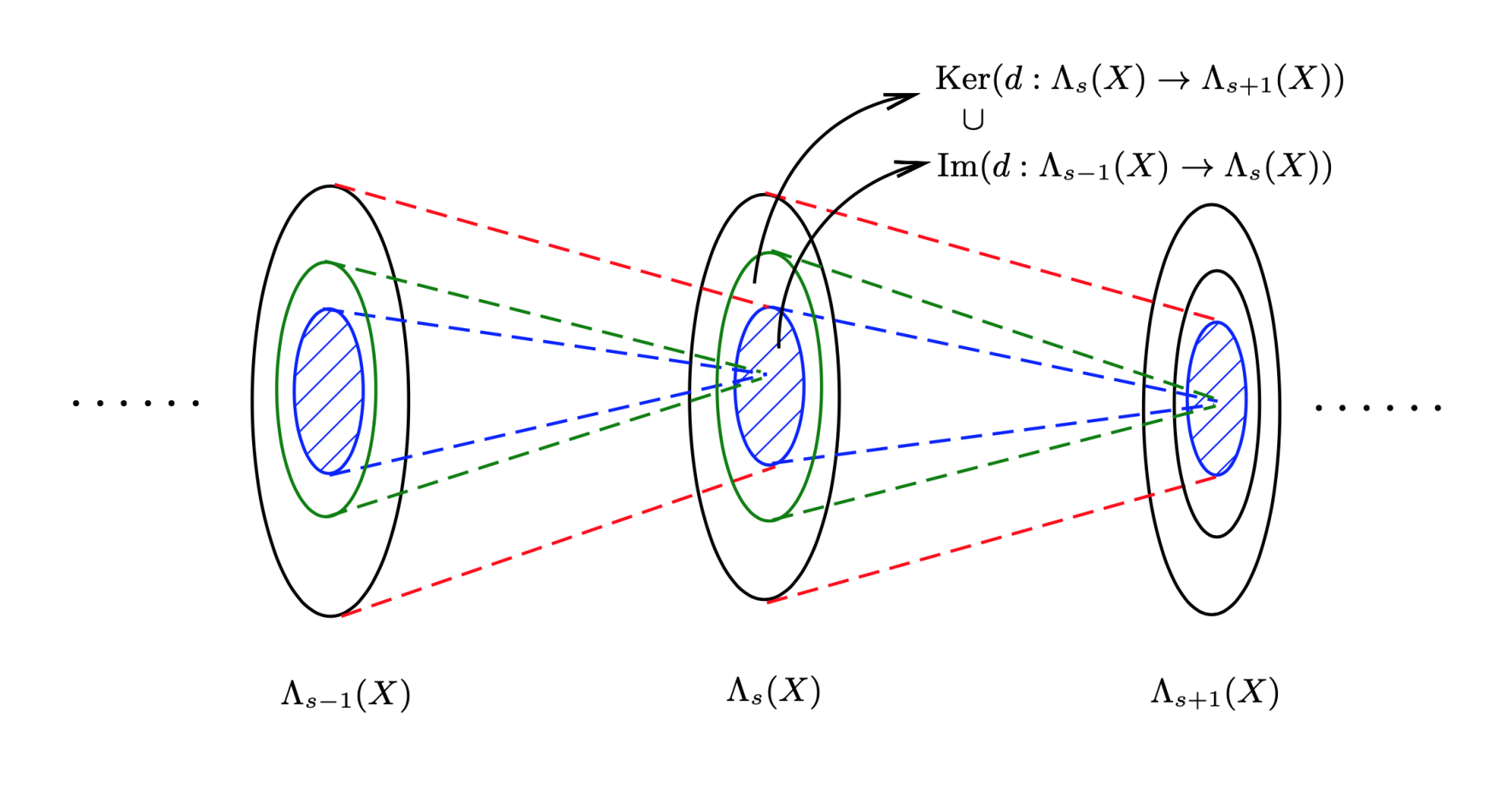## 微分形式与外微分

### 微分形式

$s$-阶全反对称张量为 $s$-形式。这些 $s$-形式（$s = 0,1\cdots$）形成外代数。

$\Lambda(T_ p X) = \bigoplus_{s=0}^{\dim (X)}\Lambda_ s (T_ p X)$

$X$ 上的每一点“光滑地”指定一个 $s$-形式，我们便得到光滑的 $s$-形式场，或简称为 $s$-形式。$X$ 上所有的 $s$-形式场的集合记为 $\Lambda_ s (X)$

$\Lambda(X) = \bigoplus_{s=0}^{\dim (X)}\Lambda_ s (X)$

$\omega$ 是一个 $s$-形式，在某个局部坐标卡下，它可以表示为：

$\omega = \frac{1}{s!}\omega_{i_1\cdots i_s}dx^{i_1}\land \cdots \land dx^{i_s}$

$\omega_{i_1\cdots i_s} = \frac{\partial \tilde{x}^{j_1}}{\partial x^{i_1}}\cdots\frac{\partial \tilde{x}^{j_s}}{\partial x^{i_s}}\tilde{\omega}_{j_1\cdots j_s}$

### 外微分

$\mathcal{O} : \Lambda(X) \rightarrow \Lambda(X)$ 是一个 $\mathbb{R}$-线性映射，使得：

$\mathcal{O}(\Lambda_ s (X)) \subset \Lambda_ {s+p} (X)$

• $\mathcal{O}$ 满足：

$\mathcal{O}(\omega \land \theta) = \mathcal{O}(\omega) \land \theta + \omega \land \mathcal{O}(\theta)$

• $\mathcal{O}$ 满足：

$\mathcal{O}(\omega \land \theta) = \mathcal{O}(\omega) \land \theta +(-1)^{\deg(\omega)} \omega \land \mathcal{O}(\theta)$

$\omega$ 是一个光滑的 $s$-形式。粗略地说，外微分算子，记为 $d$，将 $\omega$ 变成一个光滑的 $(s + 1)$-形式，并记为 $d\omega$

• $\mathbb{R}$-线性性：对任意 $\omega,\theta \in \Lambda(X), \lambda\in R$，要求

$d(\omega + \theta) = d\omega + d\theta,\quad d(\lambda\omega) = \lambda d\omega$

$d(\omega\land \theta) = d\omega\land \theta+ (-1)^ {\deg(\omega)}\omega\land d\theta$

• 幂零性（Nilpotency）

$d^2 = d \circ d = 0$

• 对任意 $f \in \mathscr{F}(X) = \Lambda_0 (X)$$df$$f$ 的微分。

$\omega = \frac{1}{s!}\omega_{i_1\cdots i_s}dx^{i_1}\land \cdots \land dx^{i_s}$

$d\omega = \frac{1}{s!}\frac{\partial \omega_{i_1\cdots i_s}}{\partial x^i}dx^{i_1}\land \cdots \land dx^{i_s}$

$\Phi : X \rightarrow Y$ 是流形 $X$ 到流形 $Y$ 的光滑映射。则 $\Phi^*$ 是由 $\Lambda (Y)$$\Lambda(X)$ 的线性映射，且可知 $\Phi^*$ 是一个线性同态。设 $\omega \in \Lambda(Y )$，则可以证明

$d(\Phi^ * \omega) = \Phi^ * (d\omega)$

$v \in \mathscr{X} (X)$，由李导数的 Leibniz 法则，我们知道：

$\mathcal{L}_ v (\omega \land \theta) = \mathcal{L}_ v \omega \land \theta + \omega \land \mathcal{L}_ v \theta$

$v \in \mathscr{X} (X)$，内乘 $i_ v$ 算子是一个线性算子：

$i_ v : \Lambda(X) \rightarrow \Lambda(X)$

$(i_v \omega)(u_ 1 ,\cdots ,u_ {s-1} ) = \omega(v,u_ 1 ,\cdots ,u_ {s-1})$

$u_i \in \mathscr{X}(X), i = 1,\cdots - 1, \omega \in \Lambda_s (X)$

$\omega \in \Lambda_ 0 (X), i_v \omega = 0$

$i_v (\omega \land \theta) = (i_ v \omega) \land \theta + (-1)^ {\deg(\omega)} \omega \land (i v \theta)$

$i^ 2_v= i_v \circ i_ v = 0$

$\mathcal{L}_v = i_ v \circ d + d \circ i_ v$

$\mathcal{L}_v \omega = d(i_ v \omega)$

### de Rham 上同调

$\{0\}\overset{d}{\longrightarrow}\Lambda_0(X)\overset{d}{\longrightarrow}\Lambda_1(X)\overset{d}{\longrightarrow}\Lambda_2(X)\overset{d}{\longrightarrow}\cdots\overset{d}{\longrightarrow}\Lambda_n(X)\overset{d}{\longrightarrow}\{0\}$• 闭形式 (closed)
$\omega \in \Lambda(X)$，若 $d\omega = 0$，则称 $\omega$ 为一个闭形式。
• 恰当形式 (exact)
$\omega \in \Lambda(X)$ 是一个微分形式 $\theta \in \Lambda(X)$ 的外微分，即 $\omega = d\theta$，则称 $\omega$ 为一个恰当形式。

de Rham 上同调群（de Rham cohomology）

\begin{aligned} &Z^ s (X) = \mathrm{Ker}(d : \Lambda _ s (X) \rightarrow \Lambda_{s+1} (X)) = \{\omega \in \Lambda_s (X)|d\omega = 0\}\\ &B^ s (X) = \mathrm{Im}(d : \Lambda_ {s-1} (X) \rightarrow \Lambda_ s (X)) = \{\omega \in \Lambda_ s (X)|\omega = d\theta\}\\ \end{aligned}

$H^ s (X) = Z^ s (X)/B^ s (X)$

$s=0$ 时，容易看出 $B^0\cong\{0\}$，而 $Z^0(X) = \mathbb{R}$，若 $X$ 为连通的光滑流形（外微分为零的光滑函数为常函数）。因此对于连通光滑流形，我们总有：

$H^0(X) = \mathbb{R}$

$X$ 是非连通流形，且有 $k$-连通部分，则它的第零个上同调群为 $H_0(X) = \mathbb{R}^k$

## 总结

• 可微结构使得我们能够在流形上每一点引入一个线性空间和余切空间，这样我们可以在流形上的每一点都引入一个张量。进一步地，我们可以研究流形上的光滑张量场。
• 到现在为止，在一个给定的光滑流形上，我们已经讨论了三种算子：李导数、外微分和内乘。它们的定义只依赖于光滑流形的微分结构。换句话说，有了微分结构之后，这些算子是可以自然定义的。在讨论它们的时候，我们不需要引入额外的数学结构。
• 微分流形是很多其他数学结构建立的平台。在接下来的笔记中，我们将引入联络和度规。它们就是在微分流形上建立的非常有用的数学结构，在广义相对论或其他一些引力理论中都是非常重要的。